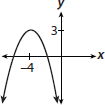# SAT Math Multiple Choice Question 931: Answer and Explanation

### Test Information

Question: 931

15. What is the range of the quadratic function whose equation is q(x) = –(x + 4)2 + 3?

• A.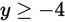• B.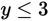• C.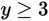• D.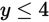Explanation:

B

Difficulty: Medium